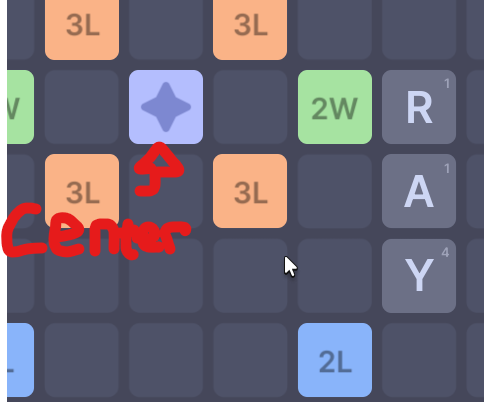# Scrabble collision math

okay so lets say i have an array containing word groups. here’s what it looks like (mockup)

``````local groups = {
{ word = "hi", positions = { Vector2.new(10, 10), Vector2.new(10, 11) }, hidden = false },
{ word = "bye", positions = { Vector2.new(13, 10), Vector2.new(13, 11), Vector2.new(13, 12) }, hidden = false },
}
``````

and lets say i have a center point. (mockup)

``````local center = Vector2.new(10, 10)
``````

how would i make sure the word groups are either building off of each other or building off of the center point? i already have the math for combining letters into groups & i validate if theyre actual english words.

but right now, a player can just build wherever they want and it’ll let them. im not greatt at math so if yall could help thatd be nice.

for context, each letter is 1,1 in size so to for example get the one to the left you’d do `letterPos - Vector2.new(1, 0)`All the open cells that are valid for placing a new word are less than one square away from the center position ( assuming diagonals are also counted as 1 away ). Expressed mathematically, we say a new word w at cell position p is valid if and only if `(p-c).Magnitude < math.sqrt(2)`, where c is the position of the center cell. Taking the square of both sides gives us the following inequality `p:dot(c) < 2`.
Note: The reason why I use sqrt(2) instead of 1 is because the distance from the center cell to a diagonal cell is sqrt(2), thus we check for a distance less than sqrt(2) rather than 1 to account for the diagonals.

i’d switch it to sqrt(1) if i didnt want diagonals right ?

1 Like